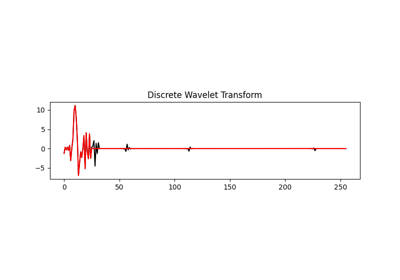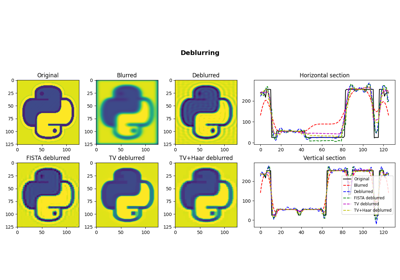# pylops.signalprocessing.DWT2D¶

class pylops.signalprocessing.DWT2D(dims, dirs=(0, 1), wavelet='haar', level=1, dtype='float64')[source]

Two dimensional Wavelet operator.

Apply 2D-Wavelet Transform along two directions dirs of a multi-dimensional array of size dims.

Note that the Wavelet operator is an overload of the pywt implementation of the wavelet transform. Refer to https://pywavelets.readthedocs.io for a detailed description of the input parameters.

Parameters: dims : tuple Number of samples for each dimension dirs : tuple, optional Direction along which DWT2D is applied. wavelet : str, optional Name of wavelet type. Use pywt.wavelist(kind='discrete') for a list of available wavelets. level : int, optional Number of scaling levels (must be >=0). dtype : str, optional Type of elements in input array. ModuleNotFoundError If pywt is not installed ValueError If wavelet does not belong to pywt.families

Notes

The Wavelet operator applies the 2-dimensional multilevel Discrete Wavelet Transform (DWT2) in forward mode and the 2-dimensional multilevel Inverse Discrete Wavelet Transform (IDWT2) in adjoint mode.

Attributes: shape : tuple Operator shape explicit : bool Operator contains a matrix that can be solved explicitly (True) or not (False)

Methods

 __init__(dims[, dirs, wavelet, level, dtype]) Initialize this LinearOperator. adjoint() Hermitian adjoint. apply_columns(cols) Apply subset of columns of operator cond([uselobpcg]) Condition number of linear operator. conj() Complex conjugate operator div(y[, niter, densesolver]) Solve the linear problem $$\mathbf{y}=\mathbf{A}\mathbf{x}$$. dot(x) Matrix-matrix or matrix-vector multiplication. eigs([neigs, symmetric, niter, uselobpcg]) Most significant eigenvalues of linear operator. matmat(X) Matrix-matrix multiplication. matvec(x) Matrix-vector multiplication. rmatmat(X) Matrix-matrix multiplication. rmatvec(x) Adjoint matrix-vector multiplication. todense([backend]) Return dense matrix. toimag([forw, adj]) Imag operator toreal([forw, adj]) Real operator tosparse() Return sparse matrix. trace([neval, method, backend]) Trace of linear operator. transpose() Transpose this linear operator.

## Examples using pylops.signalprocessing.DWT2D¶Wavelet transform05. Image deblurring